# 通俗地理解反向传播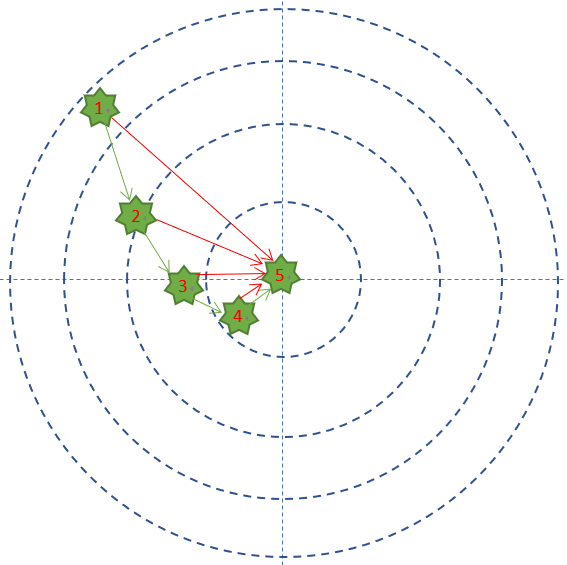• 每次试枪弹着点和靶心之间的差距就叫做误差，可以用一个误差函数来表示，比如差距的绝对值，如图中的红色线。
• 一共试枪5次，就是迭代/训练了5次的过程 。
• 每次试枪后，把靶子拉回来看弹着点，然后调整下一次的射击角度的过程，叫做反向传播。注意，把靶子拉回来看和跑到靶子前面去看有本质的区别，后者容易有生命危险，因为还有别的射击者。一个不恰当的比喻是，在数学概念中，人跑到靶子前面去看，叫做正向微分；把靶子拉回来看，叫做反向微分。
• 每次调整角度的数值和方向，叫做梯度。比如向右侧调整1毫米，或者向左下方调整2毫米。如图中的绿色矢量线。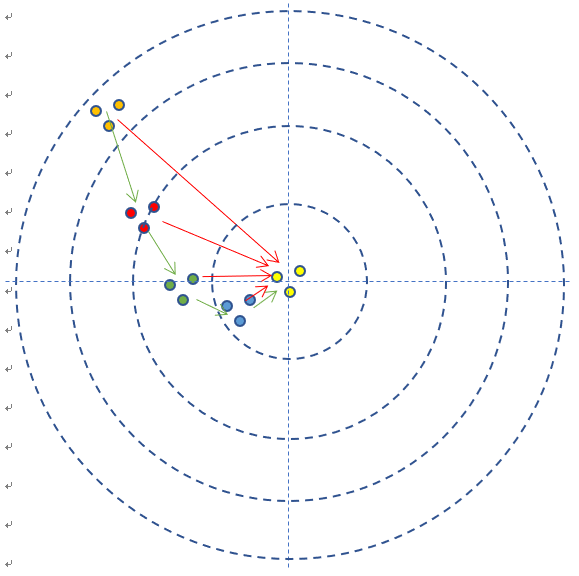• 如果每次3发子弹连发，这3发子弹的弹着点和靶心之间的差距之和再除以3，叫做损失，可以用损失函数来表示。

# 用数学概念理解反向传播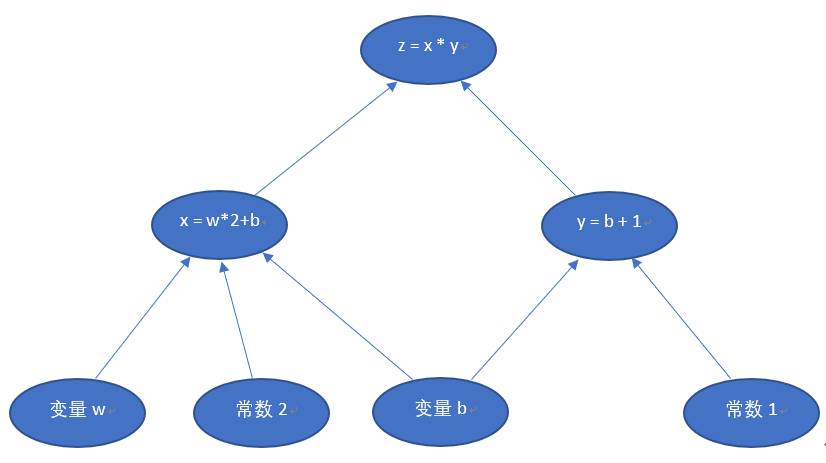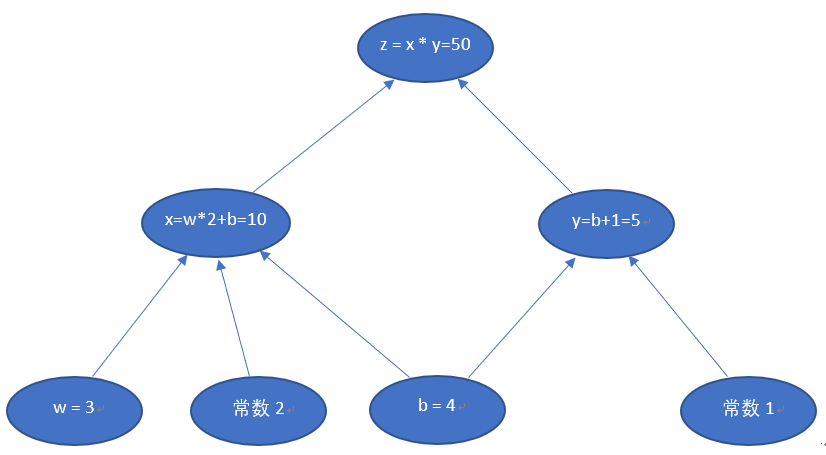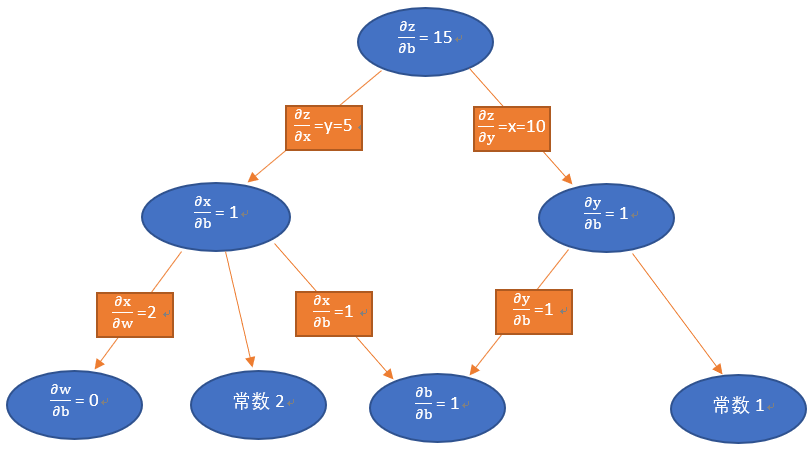$\frac{\partial{z}}{\partial{b}}=\frac{\partial{z}}{\partial{x}}*\frac{\partial{x}}{\partial{b}}+\frac{\partial{z}}{\partial{y}}*\frac{\partial{y}}{\partial{b}}=5*1+10*1=15$

$\frac{\partial{z}}{\partial{x}}=\frac{\partial{}}{\partial{x}}(x*y)=y=5$
$\frac{\partial{z}}{\partial{y}}=\frac{\partial{}}{\partial{y}}(x*y)=x=10$
$\frac{\partial{x}}{\partial{b}}=\frac{\partial{}}{\partial{b}}(w*2+b)=1$
$\frac{\partial{y}}{\partial{b}}=\frac{\partial{}}{\partial{b}}(b+1)=1$

50.5 < 51，说明y的微小变化对z的贡献比较大，这个从

$\frac{\partial{z}}{\partial{x}}=\frac{\partial{}}{\partial{x}}(x*y)=5 < \frac{\partial{z}}{\partial{y}}=\frac{\partial{}}{\partial{y}}(x*y)=10$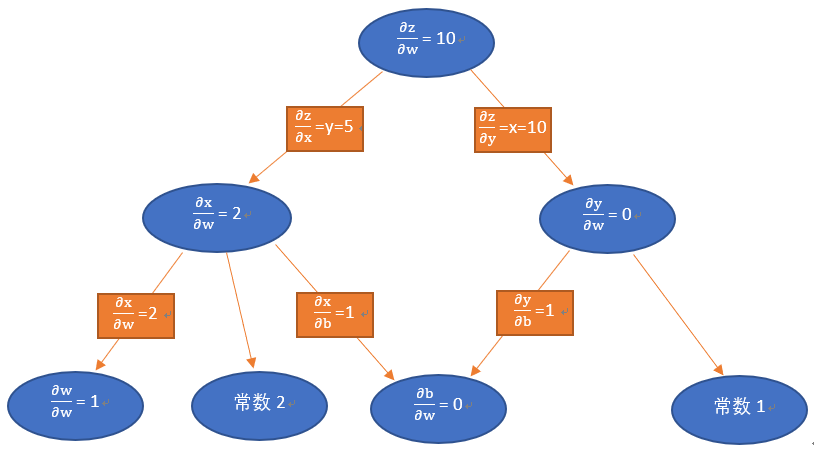w的变化和b的变化，哪一个对z的变化贡献大？从图中还可以注意到：

$\frac{\partial{z}}{\partial{b}}=15$
$\frac{\partial{z}}{\partial{w}}=10$

# 反向传播的实际计算过程（单变量）

• $w = 3$
• $b=4$
• $x = w*2+b = 10$
• $y = b+1 = 5$
• $z = x*y=50$

$\frac{\partial{z}}{\partial{b}}=\frac{\Delta{z}}{\Delta{b}}=15$

$\frac{\Delta{z}}{\Delta{b}}=15=\frac{10}{\Delta{b}} \\$

$\Delta{b} = 0.66667$

• $w = 3$
• $b=4+0.66667=4.66667$
• $x = w*2+b = 10.66667$
• $y = b+1 = 5.66667$
• $z = x*y=10.66667*5.66667=60.4445$

$\frac{\Delta{z}}{\Delta{b}}=15=\frac{-0.4445}{\Delta{b}} \\$

$\Delta{b} = -0.02963$

• $w = 3$
• $b=4.66667-0.02963=4.63704$
• $x = w*2+b = 10.63704$
• $y = b+1 = 5.63704$
• $z = x*y =10.63704*5.63704=59.96$

# 用二维平面函数说明梯度下降原理

1） 为啥用这个看上去像$y = x^2$族的函数来说明梯度下降？
2） 在最低点的左侧，梯度值是负数；在最低点的右侧，梯度值是正数。为什么说是“下降”？
3） 为什么1—>2，2—>3等等的连线不是这条曲线的切线呢，而好像是弦线？## 为何用$y = x^2$函数？

$loss = \sum_{i}(a_i - y_i) ^ 2$

## 为什么说是“梯度下降”？

“梯度下降”，刚接触这个词时，我总是往“降低难度”或“降低维度”方面去理解，因为有个“下降”的动词在里面。而实际上，“下降”在这里面的含义是“与导数相反的方向”的意思。

• 在点B上，这个函数的切线（绿色）是指向下方的（Y轴方向），所以是个负数：假设$X_B$ = 0.1, 则$y’ = 2*(0.1-1) = -1.8$
• 在F点上，切线（绿色）向上：假设$X_F$ = 1.5, 则$y’ = 2*(1.5-1) = 1$，是个正数。

$w = w – η*\Delta{w}$
$b = b – η*\Delta{b}$

• 当梯度(y')是正数时，即点F的位置，$x = x - η*1$，切线向上，x值会变小，权重值会从右侧向x=1靠近；
• 当梯度(y')是负数时，亦即点B的位置，切线向下，x值会变大$x = x - η*(-1.8) = x + η*1.8$，最终运算结果变成了加法，与切线方向相反，权重值会从左侧向x=1靠近。

## 曲线和弦线的关系？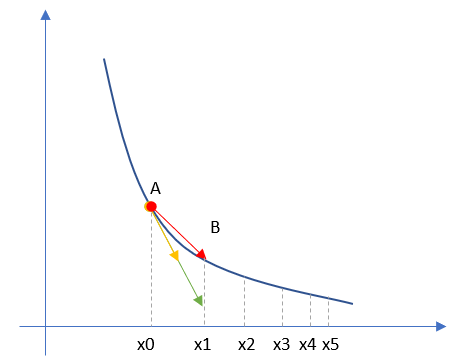1. 我们先知道了A点的切线的方向，亦即黄色的线，但是不知道长度
2. 我们有步长值η，以及梯度下降公式$X_1 = X_0 – η * dx$
3. 因为$y'_x的导数dx = 2(X-1), η = 0.1, X_0 = 0.2, 于是有X_1 = X_0–0.1*2(X_0-1) = 0.36$，这就等同于我们知道了切线的长度，亦即绿色的线的长度和方向都确定了
4. 然后我们可以画出红色的线（亦即弦线）

# 参考资料

• http://colah.github.io/posts/2015-08-Backprop/posted @ 2018-11-12 13:26 UniversalAIPlatform 阅读(...) 评论(...) 编辑 收藏